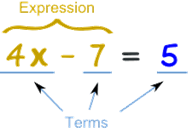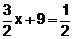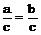# Introduction

## linear equation in one variable

“Linear” equations are the equations with just a plain variable like “x” or “y“, rather than something more complicated powers like x2 or x/y or square roots or cube roots. Linear equations are the simplest equations that one can deal with. In the linear equations will initially work with rational coefficients and rational solutions. In this chapter but in further study we will study irrational coefficient also, we shall deal with equations involving rational numbers as the coefficients and their solutions can also be rational numbers.

### Find value of ?.

If ? + 5 =7

Once you’d learned your addition facts well enough, you knew that you had to put a “2” in place of question mark. Solving equations works in much the same way, but now you have to figure out what goes into the x, instead of what goes into place of question mark. But in this class, the equations can be much more complicated, and therefore the methods you’ll use to solve the equations will be a bit more advanced. An open sentence containing the sign of equality is called an equation

 eg : a) x - 2 = 4 b) 3x - 2 = x - 4 c) 3x = 12 d) x/3 = 6

In general, to solve an equation for a given variable, you need to “undo” whatever has been done to the variable. You do this in order to get the variable by itself; in technical terms, you are “isolating” the variable. This results in “(variable) equals (some number)”, where (some number) is the answer they’re looking for. For instance:

Solve x + 9 = -2

I want to get the x by itself; that is, I want to get “x” on one side of the “equals” sign, and some number on the other side. Since I want just x on the one side, this means that I don’t like the “plus nine” that’s currently on the same side as the x. Since the 9 is added to the x, I need to subtract to get rid of it. That is, I will need to subtract a 9 from the x in order to “undo” having added a 9 to it.

X + 9 = -2

X + 9 - 9 = – 2 – 9 = – 11

#### A number which when replaced for the variable of an equation makes it a true statement is called 'root' of the equation.

Eg : x + 7 = 10 3 + 7 = 10 10 = 10 (LHS = RHS)

x = 3 is the root of the equation x + 7 = 10

Equation is define as – A statement of equality which contains one or more unknown quantity or

variable (literals) is called an equation.

An equation says that two things are the same, using mathematical symbols.

An equal sign (=) is used### LINEAR EQUATION

An equation involving only linear polynomials is called a linear equation.

Ex. 3x - 2 = 7,are linear equation in one variable, because the highest power of the variable in each equation is one whereas the equations3x2 - 2x + 1 = 0, y2 - 1 = 8 are not linear equations, because the highest power of the variable in each equations, because the highest power of the variable equation is not one.

### SOLUTION OF A LINEAR EQUATION SOLUTION

A value of the variable which when substituted for the variable in an equation makes L.H.S. = R.H.S. is said to satisfy the equation and is called a solution or a root of the equation.

In other words, a value of the variable which makes the equation a true statement is called a solution or a root of the equation.

### THINGS TO REMEMBER

a) Addition property : If the same number is added to both sides of an equality, the equality is not altered.

If x = y, then x + z = y + z where x, y, z are any three numbers.

b) Subtraction property : If the same number is subtracted from both sides of an equality, the equality is not altered.

If x = y, then x - a = y - a where x, y, a are any three numbers.

c) Multiplication property : If both sides of an equality are multiplied by the same number, the equality is not altered.

If a = b, then ac = bc, where a, b, c are any three numbers.

d) Division property : If both sides of an equality are divided by the same non zero number, then the equality is not altered.

If a =b , thenwhere a, b, c are any three numbers and c is non zero quantity.

To score More in your class 7 refer NCERT solutions for class 7 Maths